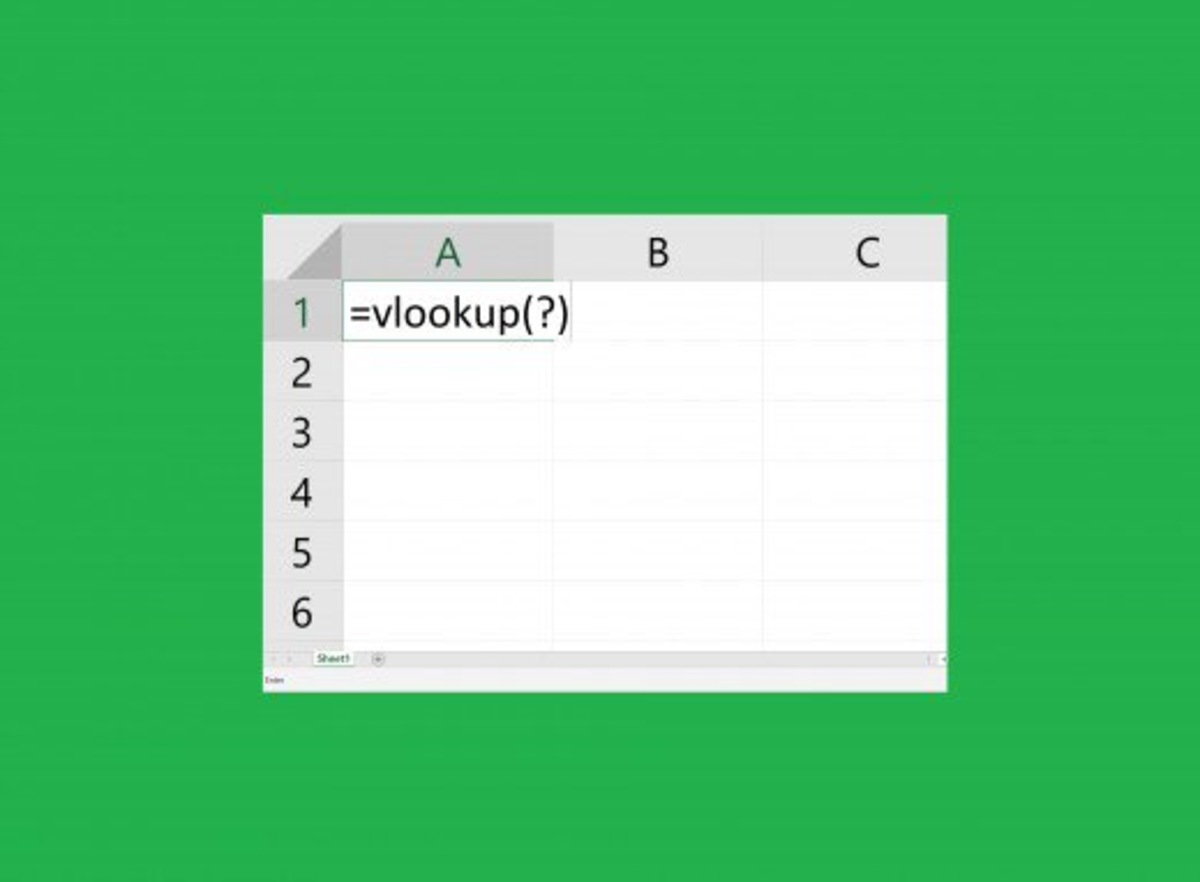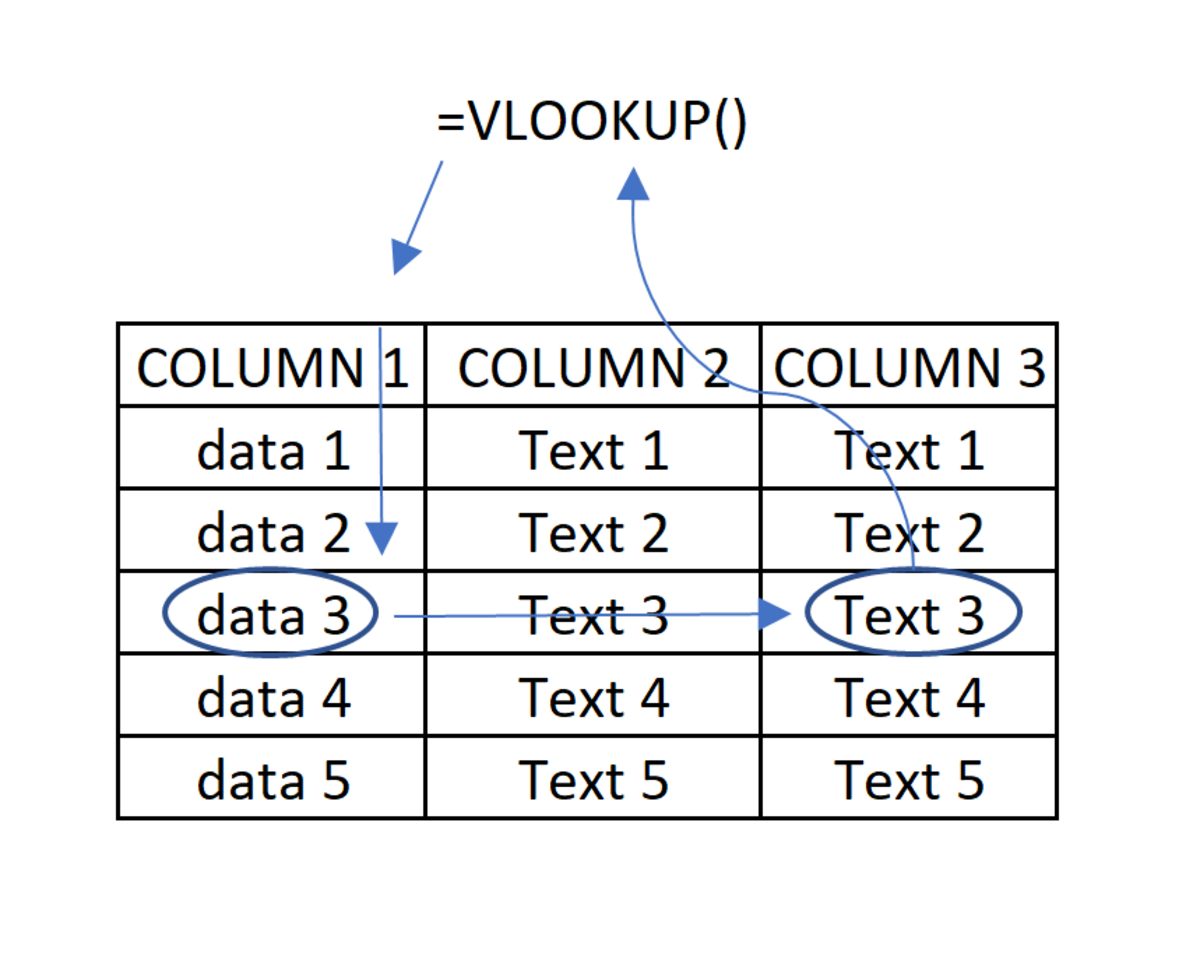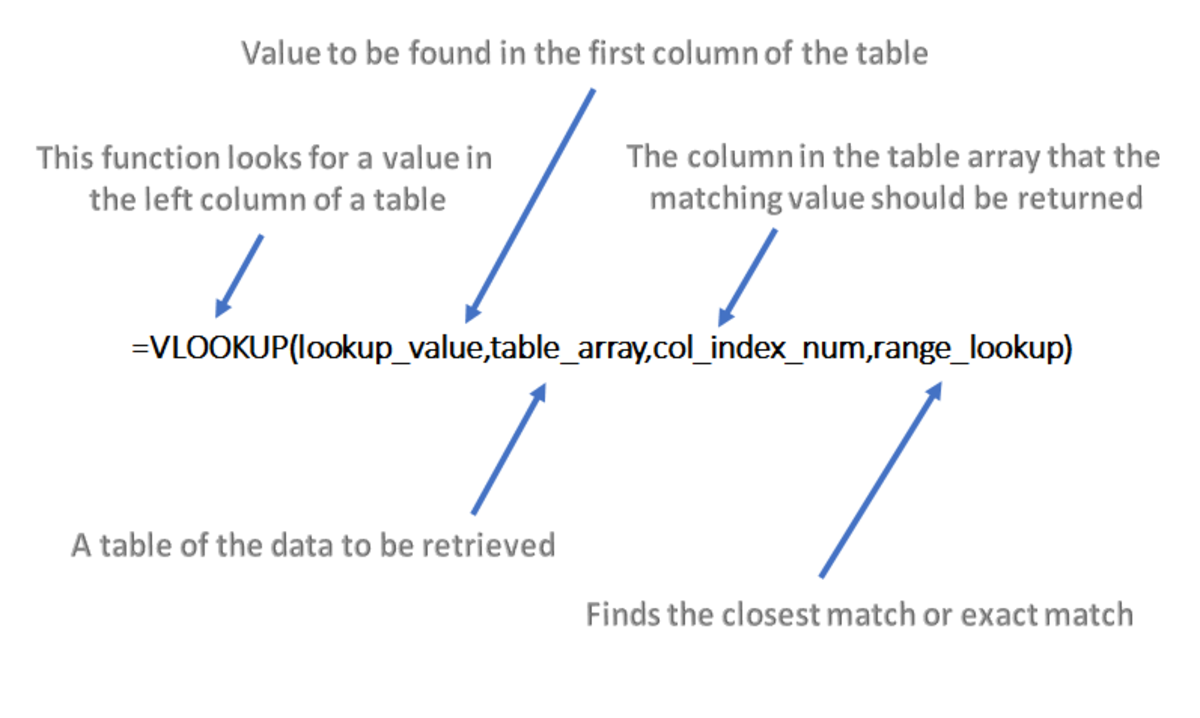How to Use the VLOOKUP Function in MS Excel 2016

James likes to learn about technology and share what he learns through his articles.The VLOOKUP function could be one of Excel’s most powerful functions. To many the VLOOKUP may be one of the lesser understood or even known Excel functions. This function is worth practicing since it has many useful applications in business.

How to Use VLOOKUP in Excel 2016

The VLOOKUP function is a powerful Microsoft Office tool and can be utilized for many applications. In this article, I give some basic instruction on how to use this function as a lookup/reference tool for displaying information. This tutorial is conducted in Excel 2016 but applies to version Excel 2007 and higher. Don’t be alarmed if you are a beginner because using VLOOKUP in this example is very basic. Let’s see how it works.

How VLOOKUP Works

The VLOOKUP function is very useful as it can display information by using a reference value in the function. The V in VLOOKUP stands for vertical. So, when this function is used a piece of selected data from a column (vertically aligned data) is referenced. After a match is made, data is selected from a column that is preselected in the function and is returned to display in the cell that contains the function. A simplified illustration of the function process can be seen below.

Another function that is like the VLOOKUP function is the HLOOKUP function. The H in HLOOKUP stands for horizontal. The HLOOKUP has the same premise except it references information in the first row of the array to display information found in other rows.

VLOOKUP ProcessThe VLOOKUP function can be easily understood from this illustration. A piece of data is referenced in the first column of a table array. A match is made, then text is returned to display data that comes from a preselected a column.

Setting Up the Function

The structure of the VLOOKUP formula is shown below:

=VLOOKUP(lookup_value,table_array,col_index_num,range_lookup)

The first value is the lookup value. This is the value that Excel is going to search for in the range. The lookup value can be a number, reference or formula. When the value is found Excel will return any data that lies in a column to the right of this data.

The next value is table array. This table contains lookup value and any data that may be returned for the VLOOKUP function. This value may be referred to as the lookup range or the lookup table.

The next value in the function column in index. This is the column that contains that value that you want to match to return another value. So, if the lookup value in located in the column index, then data from another part of the data can be returned. This may be a little difficult to understand, but when you see it in an example, everything will make sense.

The range lookup is going to be stated as a false or true statement. A false statement will make an exact match when referencing the lookup table, and a true statement will look for an approximate match.

Diagram of the VLOOKUP FunctionThe diagram highlights what each element of the function is for.

VLOOKUP Example

Let's start out by looking at a simple example so you can get a visual of how this function works. In the illustration below on the left side, there is a table of new hires for JR Wheel Company. Two of the columns in the table are related to the salary of new employees and are empty.

To the right, this table is another table that will serve as a reference so that the starting salary can be looked up based on the salary rating. The lookup table contains all the data that is missing from the employee table. It is the job of human resources to put all the employee records (including the salary rating) then the salary will appear based on the rating entered.

To return the proper starting salary, the same VLOOKUP function is used in each starting salary cell. The function will populate the starting salary column based on data that in the salary rating column.

First Step to Inserting a Function

Functions can be typed in manually, but here we will insert a function. The first thing that needs to be done to insert a function is to click in the cell where you need the function to appear. Next, click on the function tab, then click on the insert function button.

Find the Function

The insert function window appears. The function name and is typed in here and Go is pressed. Next, the user selects the correct function from the list and clicks OK.

Second Step to Inserting a Function

Each parameter of the function needs to be entered into this next window. Details on each parameter are listed below.

Lookup_value

This is going to be the salary index for this record. The cell E6 is chosen because it is the salary index for John Sherwood in the first record.

Table_array

The table array is the table where the data is being looked up. The table array chosen is I6:J10. This is all the data in the lookup table excluding the column headers.

Col_index_num

This is the column that will display data in where the function is inputted. Note that the column being referenced for the lookup value is always column 1. The column index needs to be column 2,3,4, or any column with data that you need to display that is past column 1. In this example, 2 is chosen and the only possible piece of data that can be displayed.

Range_lookup

This is set to true when you are looking for an approximate match and set at false when looking for an exact match. Since the salary rating is a discrete variable, the false will be used to find an exact number. In this situation either can be used and will return the same salary value.

Note that each time you click into a field where one of these parameters belongs a tip comes on the screen to your tips.

After each parameter is entered click the OK button.

Remove the Error Message

The salary rating was left out on purpose to show an error can occur. To be useful, this function will be added before any other data is added so it can serve its purpose of returning a value automatically. Below shows the standard function used that created the error.

=VLOOKUP(E6,I6:J10,2,FALSE)

To remove the error the IFERROR function to be used and can be seen added on the VLOOKUP function here.

=IFERROR(VLOOKUP(E6,I6:J10,2,FALSE)," ")

The IFERROR statement takes the existing function and prints a space (indicated by the " " ) if the function returns an error message.

Fix the Position of the Array

To be able to drag the formula to copy to other cells the table array of the formula needs to be fixed by adding dollar signs to the rows and columns of the array. This can be done manually or by selecting the array and tapping the F4 key.

Copy the Function

Now use the fill handle to the copy the formula. Each corresponding salary should appear when the salary rating is added to the table.